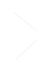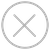TensorSpace.jsTensorSpace ConverterTensorFlow

tf.keras model

``````\$ tensorspacejs_converter \
--input_model_from="tensorflow" \
--input_model_format="tf_keras" \
--output_layer_names="layer1Name,layer2Name,layer3Name" \
./PATH/TO/MODEL/xxx.h5 \
./PATH/TO/SAVE/DIR``````
tf.keras (separated)

``````\$ tensorspacejs_converter \
--input_model_from="tensorflow" \
--input_model_format="tf_keras_separated" \
--output_layer_names="layer1Name,layer2Name,layer3Name" \
./PATH/TO/MODEL/xxx.json,./PATH/TO/MODEL/eee.h5 \
./PATH/TO/SAVE/DIR``````
frozen model

``````\$ tensorspacejs_converter \
--input_model_from="tensorflow" \
--input_model_format="tf_frozen" \
--output_layer_names="layer1Name,layer2Name,layer3Name" \
./PATH/TO/MODEL/xxx.pb \
./PATH/TO/SAVE/DIR``````
saved model

``````\$ tensorspacejs_converter \
--input_model_from="tensorflow" \
--input_model_format="tf_saved" \
--output_layer_names="layer1Name,layer2Name,layer3Name" \
./PATH/TO/SAVED/MODEL/FOLDER \
./PATH/TO/SAVE/DIR``````Keras

Combined .h5

``````\$ tensorspacejs_converter \
--input_model_from="keras" \
--input_model_format="topology_weights_combined" \
--output_layer_names="layer1Name,layer2Name,layer3Name" \
./PATH/TO/MODEL/xxx.h5 \
./PATH/TO/SAVE/DIR``````
Separated .json & .h5

``````\$ tensorspacejs_converter \
--input_model_from="keras" \
--input_model_format="topology_weights_separated" \
--output_layer_names="layer1Name,layer2Name,layer3Name" \
./PATH/TO/MODEL/xxx.json,./PATH/TO/MODEL/eee.h5 \
./PATH/TO/SAVE/DIR``````TensorFlow.js

``````\$ tensorspacejs_converter \
--input_model_from="tfjs" \
--output_layer_names="layer1Name,layer2Name,layer3Name" \
./PATH/TO/MODEL/xxx.json \
./PATH/TO/SAVE/DIR``````